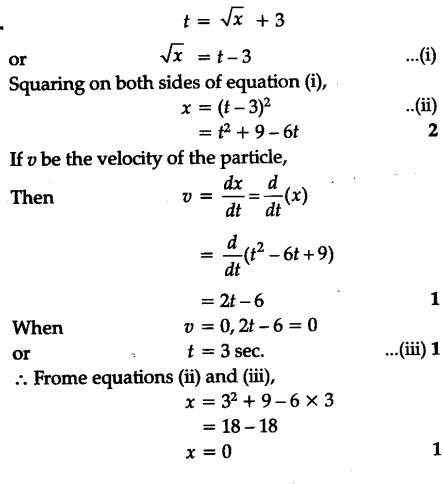# The displacement of a particle moving in one dimension under the action of a constant force is

#1

The displacement of a particle moving in one dimension under the action of a constant force is related to the time t by the equation t = square root of x + 3, where x is in metre and t is in second. Find the displacement of the particle when its velocity is zero

#2#3

The velocity can not be zero if the force is being exerted and there is some displacement due to force, so the answer is that velocity can’t be zero

#4

Preparing for school competitive examinations and want to have an edge over all, Read about the latest happenings in NTSC, KVPY, NSO examinations at Exam Bazaar- The complete guide to Examinations, Study materials and Latest trends on Exam patterns.